# Combining Normal Maps in Photoshop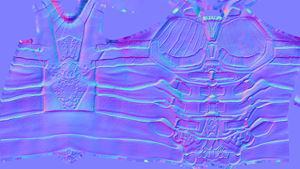This tutorial will outline the best way I've found to 'add' two normal maps in photoshop. This method specifically adds two normal maps it doesn't average, subtract or multiply. Basic Concept:

To combine two normals together (normal map A to normal map B) you need to add the positive components and subtract the negative components.

Anatomy of a normal map:

The typical RGB normal map (not a DXT5) is broken up into 3 channels:

Red Channel which represents the X values of the normals in a -1 to 1 range Green Channel which represents the Y values of the normals in a -1 to 1 range Blue Channel which represents the Z values of the normals in a 0 to 1 range

Notice how the blue channel doesn't have any negative values, this is because the Z value of a normal cannot be negative – it must point outward. If you load up a RGB normal map image into photo shop and goto "image|adjustments|levels…" you'll see something that looks like this: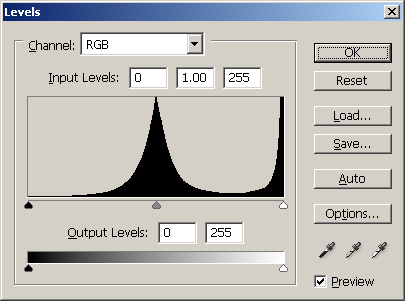This is a combined view of all three channels. Under each channel you can see the positive and negative values as mentioned above: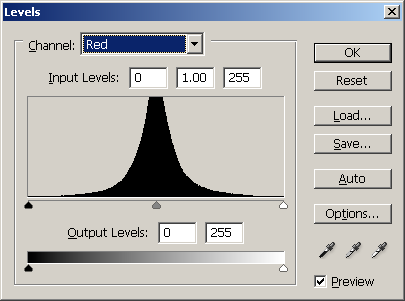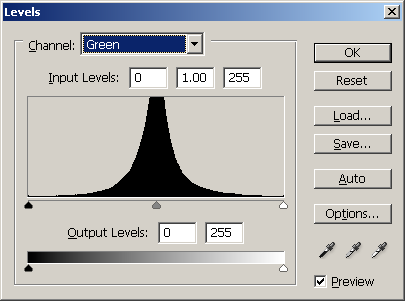So from left to middle represents the quantity of negative values on X & Y (Red & Green respectively) contained in the image. From middle to right represents the quantity of postive values on X & Y contained in the image. Normal X -1 = Red Channel 0 Normal X 0 = Red Channel 127 Normal X 1 = Red Channel 255 Normal Y -1 = Green Channel 0 Normal Y 0 = Green Channel 127 Normal Y 1 = Green Channel 255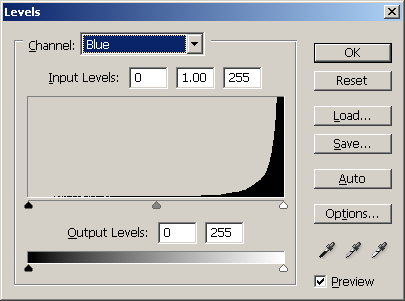However the blue channel left to right represents the quantity of positive values on Z contained in the image. Normal Z -1 = Not represented Normal Z 0 = Blue Channel 0 Normal Z 1 = Blue Channel 255 Since the default Z (or blue) value for a normal is 1 (or 255) this channel can only subtract from this value therefore it cannot make the Z value greater then 1.

What does this all mean?

Basically since we are representing normal data using an image format and that not all three channels are storing data in the same way we need to make sure we handle each channel to take this into account. Most importantly though this also identifies that each channel contains both negative and positive values represented in a positive range. Which means any technique that just Adds, Subtracts or Multiplies will result in incorrect results. Default Normal = (X 0, Y 0, Z 1) = (R 127, G 127, B 255) This default normal is the base normal for the polygon so any texel with the RGB normal value of 127,127,255 will use the polygons normal.

Part I – Break image into positive and negative values:

Note:

Positive Values:

Red 128 -> 255 Green 128 -> 255 Blue NA

Negative Values:

Red 0 -> 128 Green 0 -> 128 Blue 0 -> 255

1. Duplicate Normal Map A and rename it to "NormalMapA_Add"
2. Rename Normal Map A to "NormalMapA_Subtract"

3. Set the Red Input channels to '128' '1.00' '255'
4. Set the Red Output Levels to '128' '255'
5. Set the Green Input Channels to '128' '1.00' '255'
6. Set the Green Output Levels to '128' '255'
7. Set the Blue Output Levels to '0' '0' (this mutes the blue channel)

Remove positive values from "NormalMapA_Subtract"

1. Select "NormalMapA_Subtact" layer
3. Set the Red Input channels to '0' '1.00' '127'
4. Set the Red Output Levels to '0' '127'
5. Set the Green Input Channels to '0' '1.00' '127'
6. Set the Green Output Levels to '0' '127'

NB: Don't make any changes to the Blue channel.

Part II – Convert layers to value offsets :

Note: In order to get the offset for both the add and subtract layers we need to find out how much they deviate from the default value. As stated above the default normal vector is <0, 0, 1> .

Default Vector Value:

X 0 = R 127 Y 0 = G 127 Z 1 = B 255

Therefore we need to add this to the subtract layer and subtract it from the add layer to get the offset for each. To do this we can use a photoshop process called 'channel mixer'. Convert "NormalMapA_Subtract" to offset values

1. Select "NormalMapA_Subtact" layer
3. In 'Output Channel Red' Set the
• Red to -100%
• Green to 0%
• Blue to 0%
4. Set the constant to +50%
5. In 'Output Channel Green' Set the
• Red to 0%
• Green to -100%
• Blue to 0%
6. Set the constant to +50%
7. In 'Output Channel Blue' Set the
• Red to 0%
• Green to 0%
• Blue to -100%
8. Set the constant to 100%

3. In 'Output Channel Red' Set the
• Red to +100%
• Green to 0%
• Blue to 0%
4. Set the constant to -50%
5. In 'Output Channel Green' Set the
• Red to 0%
• Green to +100%
• Blue to 0%
6. Set the constant to -50%
7. In 'Output Channel Blue' Set the
• Red to 0%
• Green to 0%
• Blue to +100%
8. Set the constant to 0%

Part III – Set the layer blend types :

2. Set the layers blend mode to 'Linear Dodge'
3. Select "NormalMapA_Subtact" layer
4. Set the layers blend mode to 'Difference'

And thats it! You can either flatten the layers or leave them as blend layers. I typically add the two layers into their own folder (group) which I can then flatten when needed. You can download this whole process as an action here.

## 6 thoughts on “Combining Normal Maps in Photoshop”

1.simon says:

Great help for me.Thank you!

2.Rodrigo says:

Thanks for this information. Very very good.

3.Anonymous says:

Hee Rod Green
Thanks for this great tutorial and the action you posted. It really is helpfull in understanding how normals actually works.

4.Juha says:

Hey!
Thanks for this helpful tutorial and the action works perfectly!

5.koy says:

very nice tool, works perfectly sir.
i am grateful, i so often see poorly combined maps, this should be on everyones radar.